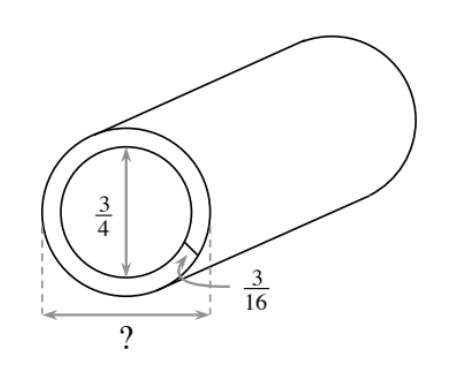### Home > ACC7 > Chapter 9 Unit 6 > Lesson CC2: 9.1.2 > Problem9-38

9-38.

Stuart is a plumber working on the Garcia family’s house. He is working with PVC pipe that has an internal diameter of $\frac { 3 } { 4 }$ inch. The thickness of the pipe is $\frac { 3 } { 16 }$ inch. What is the exterior (outside) diameter of the pipe?The diameter of the exterior of the pipe is equal to the interior diameter plus two times the thickness of the pipe, because from one side of the pipe to the other, the thickness of the pipe is included two times.

$\frac{3}{4}+\frac{3}{16}+\frac{3}{16}=\text{outside diameter}$

Find a common denominator and add.

$1\frac{1}{8}$